# Central Science: Chapter 21 Flashcards

Set Details Share
created 3 years ago by Chemistry4Life
2,156 views
updated 3 years ago by Chemistry4Life
College: First year, College: Second year, College: Third year, College: Fourth year
Subjects:
chemistry
Page to share:
Embed this setcancel
COPY
code changes based on your size selection
Size:
X

1

All atoms of a given element have the same ________.
A) mass number
B) number of nucleons
C) atomic mass
D) number of neutrons
E) atomic number

E

2

Atoms containing radioactive nuclei are called ________.
C) nucleons
D) nuclides

B

3

What happens to the mass number and the atomic number of an element when it undergoes beta decay?
A) Neither the mass number nor the atomic number changes.
B) The mass number decreases by 4 and the atomic number decreases by 2.
C) The mass number does not change and the atomic number increases by 1.
D) The mass number does not change and the atomic number decreases by 2.
E) The mass number increases by 2 and the atomic number increases by 1.

C

4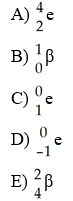Which one of the following is a correct representation of a beta particle?

D

5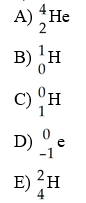Which one of the following is a correct representation of an alpha particle?

A

6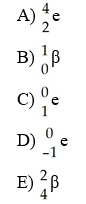Which one of the following is a correct representation of a positron?

C

7

Which one of the following processes results in an increase in the atomic number?
A) gamma emission
B) positron emission
C) beta emission
D) alpha emission
E) corrosion

C

8

Of the following processes, which one changes the atomic number?
A) alpha emission
B) beta emission
C) electron capture
D) positron emission
E) All of these processes change the atomic numbers.

E

9

What radioactive element is used to diagnose medical conditions of the heart and arteries?
A) cobalt-60
B) thallium-201
E) thorium-234

B

10

In what type of radioactive decay does the atomic number of the product increase by one?
A) alpha
B) beta
C) gamma
D) positron emission
E) electron capture

B

11

Which type of radioactive decay results in no change in mass number and atomic number for the starting nucleus?
A) alpha
B) beta
C) positron emission
D) electron capture
E) gamma

E

12

Alpha decay produces a new nucleus whose ________ than those respectively of the original nucleus.
A) atomic number is 2 less and mass number is 2 less
B) atomic number is 1 less and mass number is 2 less
C) atomic number is 2 less and mass number is 4 less
D) atomic number is 2 more and mass number is 4 more
E) atomic number is 2 more and mass number is 2 less

C

13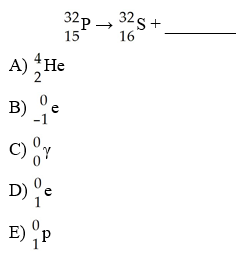What is the missing product from this reaction?

B

14

What is the atomic number of a neutron?
A) 3
B) 1
C) 2
D) 0
E) 4

D

15

What happens to the mass number and the atomic number of an element when it emits gamma radiation?
A) The mass number remains unchanged while the atomic number decreases by one.
B) The mass number decreases by four and the atomic number decreases by two.
C) The mass number increases by four and the atomic number increases by two.
D) The mass number remains unchanged while the atomic number increases by one.
E) The mass number and atomic numbers remain unchanged.

E

16

Atoms with the same atomic number and different mass numbers ________.
A) do not exist
B) are isomers
C) are isotopes
D) are allotropes
E) are resonance structures

C

17

How many radioactive decay series exist in nature?
A) 0
B) 1
C) 2
D) 3
E) 10

D

18

At approximately what number of protons, or neutrons, does the 1:1 ratio of protons to neutrons start to produce unstable nuclei?
A) 10
B) 20
C) 30
D) 50
E) 80

B

19

Carbon-11 decays by ________.
A) alpha emission
B) beta emission
C) positron emission
D) photon emission
E) neutron capture

C

20

The formation of krypton from rubidium decay is a result of ________.
A) alpha emission
B) beta emission
C) positron emission
D) electron capture
E) neutron capture

D

21

The mode of decay of 32P is ________.
A) alpha emission
B) beta emission
C) positron emission
D) electron capture
E) neutron capture

B

22

The belt of nuclear stability ends with the element ________.
B) polonium
D) astatine
E) bismuth

E

23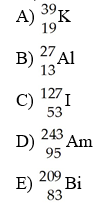Which of these nuclides is most likely to be radioactive?

D

24

What is required for a nuclear transmutation to occur?
A) very high temperature
B) a corrosive environment
C) a particle to collide with a nucleus or neutron
D) spontaneous nuclear decay
E) gamma emission

C

25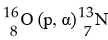In the nuclear transmutation, [see image], what is the bombarding particle?
A) an alpha particle
B) a beta particle
C) a gamma photon
D) a proton
E) a phosphorus nucleus

D

26

Cobalt-60 is produced by a three reaction process involving neutron capture, beta-emission, and neutron capture. The initial reactant in the production of cobalt-60 is ________.
A) 59Co
B) 56Fe
C) 58Fe
D) 61Co
E) 60Fe

C

27

The product of the nuclear reaction in which 28Si is subjected to neutron capture followed by alpha emission is ________.
A) 31S
B) 33S
C) 23Mg
D) 25Mg
E) 25Al

D

28

Transuranium elements have atomic numbers greater than ________.
A) 90
B) 91
C) 92
D) 93
E) 94

C

29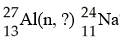What is emitted in the nuclear transmutation [see image]?
A) an alpha particle
B) a beta particle
C) a neutron
D) a proton
E) a gamma photon

A

30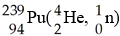In the nuclear transmutation represented by [see image]? what is the product?
A) uranium-242
B) curium-245
C) curium-242
D) uranium-245
E) uranium-243

C

31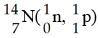In the nuclear transmutation represented by [see image]?, what is the emitted particle?
A) neutron
B) proton
C) positron
D) alpha particle
E) electron

B

32What is the product for the nuclear transmutation represented by [see image]?
A) carbon-12
B) carbon-16
C) nitrogen-16
D) carbon-14
E) nitrogen-15

D

33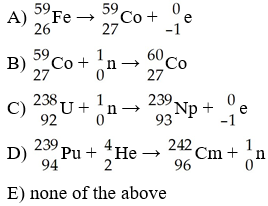Which one of the following requires a particle accelerator to occur?

D

34

Bombardment of uranium-238 with a deuteron (hydrogen-2) generates neptunium-237 and ________ neutrons.
A) 1
B) 2
C) 3
D) 4
E) 5

C

35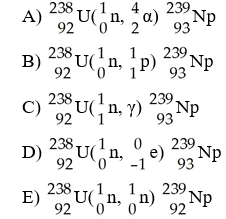The transmutation in which neptunium-239 is produced via bombardment of uranium-238 with a neutron is represented by ________.

D

36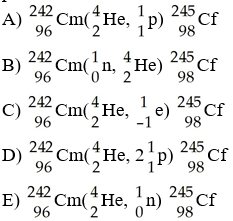The transmutation in which a curium-242 nucleus is bombarded with an alpha particle to produce a californium-245 nucleus is represented by ________.

E

37

Which one of the following can be done to shorten the half-life of the radioactive decay of uranium-238?
A) freeze it
B) heat it
C) convert it to UF6
D) oxidize it to the +2 oxidation state
E) none of the above

E

38

The beta decay of cesium-137 has a half-life of 30.0 years. How many years must pass to reduce a 25 mg sample of cesium 137 to 8.7 mg?
A) 46
B) 32
C) 3.2
D) 50
E) 52

A

39

The half-life of 218Po is 3.1 minutes. How much of a 155 gram sample remains after 0.40 hours?
A) 0.00067 g
B) 0.0072 g
C) 0.72 g
D) 0.0047 g
E) none of the above

C

40

Cesium-131 has a half-life of 9.7 days. What percent of a cesium-131 sample remains after 60 days?
A) 100
B) 0
C) 1.4
D) 98.6

C

41

The half-life for beta decay of strontium-90 is 28.8 years. A milk sample is found to contain 10.3 ppm strontium-90. How many years would pass before the strontium-90 concentration would drop to 1.0 ppm?
A) 92.3
B) 0.112
C) 186
D) 96.9
E) 131

D

42

The half-life of carbon-11 is 20.3 minutes. How much of a 100.0 mg sample remains after 1.50 hours?
A) 8.48 mg
B) 4.63 mg
C) 12.9 mg
D) 22.6 mg
E) 7.70 mg

B

43

The half-life of 131I is 0.220 years. How much of a 500.0 mg sample remains after 24 hours?
A) 496 mg
B) 560 mg
C) 219 mg
D) 405 mg
E) 337 mg

A

44

The half-life of 223Ra is 11.4 days. How much of a 200.0 mg sample remains after 600 hours?
A) 0.219 mg
B) 21.9 mg
C) .0302 mg
D) 43.8 mg
E) 6.04 mg

D

45

The half-life of 222Rn is 3.80 days. If a sample contains 36.0 g of Rn-222, how many years will it take for the sample to be reduced to 1.00 mg of Rn-222?
A) 19.7
B) 0.1575
C) 8.53
D) 0.0234
E) none of the above

B

46

The carbon-14 dating method can be used to determine the age of a ________.
B) papyrus scroll
D) clay pot
E) rock

B

47

The basis for the carbon-14 dating method is that ________.
A) the amount of carbon-14 in all objects is the same
B) carbon-14 is very unstable and is readily lost from the atmosphere
C) the ratio of carbon-14 to carbon-12 in the atmosphere is a constant
D) living tissue will not absorb carbon-14 but will absorb carbon-12
E) All of the above are correct.

C

48

Pb has a half-life of 22.3 years and decays to produce 206Hg. If you start with 7.50 g of 210Pb, how many grams of 206Hg will you have after 17.5 years?
A) 4.35
B) 3.15
C) 3.09
D) 0.0600
E) 1.71

B

49

The half-life of a radionuclide ________.
A) is constant
B) gets shorter with passing time
C) gets longer with passing time
D) gets shorter with increased temperature
E) gets longer with increased temperature

A

50

The curie is a measure of the ________.
A) number of disintegrations per second of a radioactive substance
B) total energy absorbed by an object exposed to a radioactive source
C) lethal threshold for radiation exposure
D) number of alpha particles emitted by exactly one gram of a radioactive substance
E) None of the above is correct.

A

51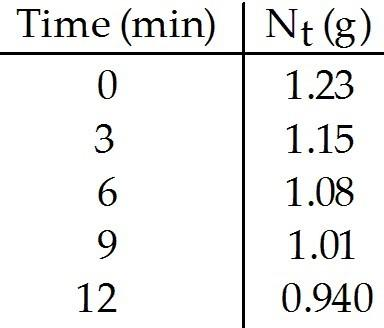What is the rate constant (in min-1) for the decay of this radionuclide?
A) 45
B) 32
C) 0.024
D) 0.032
E) 0.022

E

52What is the half-life (in min) of this radionuclide?
A) 0.024
B) 0.022
C) 32
D) 0.032
E) 45

C

53

Cesium-137 undergoes beta decay and has a half-life of 30.0 years. How many beta particles are emitted by a 14.0-g sample of cesium-137 in three minutes?
A) 6.1 × 1013
B) 6.2 × 1022
C) 8.4 × 1015
D) 1.3 × 10-8
E) 8.1 × 1015

E

54

What is a phosphor?
A) an oxide of phosphorus
B) a substance that thermally reduces to phosphorus
C) a bioluminescent substance
D) a substance that emits light when excited by radiation
E) an alkali metal phosphide

D

55

Which one of the following devices converts radioactive emissions to light for detection?
A) Geiger counter
B) photographic film
C) scintillation counter
E) none of the above

C

56

Which one of the following is used as a radiotracer to study blood?
A) iron-59
B) technetium-99
C) sodium-23
D) iodine-131
E) phosphorus-32

A

57

Which one of the following is true?
A) Some spontaneous nuclear reactions are exothermic.
B) Some spontaneous nuclear reactions are endothermic.
C) All spontaneous nuclear reactions are exothermic.
D) There is no relationship between exothermicity and spontaneity in nuclear reactions.
E) All spontaneous nuclear reactions are endothermic.

C

58

The mass of a proton is 1.673 × 10-24 g. The mass of a neutron is 1.675 × 10-24 g. The mass of the nucleus of an 56Fe atom is 9.289 × 10-23 g. What is the nuclear binding energy (in J) for a 56Fe nucleus? (c = 3.00 x 108 m/s)
A) 2.57 × 10-16
B) 7.72 × 10-8
C) 8.36 × 10-9
D) 7.72 × 10-11
E) 6.07 × 106

D

59

When two atoms of 2H are fused to form one atom of 4He, the total energy evolved is 3.83 x 10-12J. What is the total change in mass (in kg) for this reaction? (c = 3.00 x 108 m/s)
A) 1.28 × 10-17
B) 4.26 × 10-26
C) 3.45 × 108
D) 1.15
E) 4.26 × 10-29

E

60

The mass of a proton is 1.00728 amu and that of a neutron is 1.00867 amu. What is the binding energy (in J) of a 60 27Co nucleus? (The mass of a cobalt-60 nucleus is 59.9338 amu. Speed of light = 3.00 × 108 m/s.)
A) 2.74 × 10-19
B) 9.12 × 10-28
C) 4.94 × 10-13
D) 8.20 × 10-11
E) 2.74 × 10-16

D

61

The mass of a proton is 1.00728 amu and that of a neutron is 1.00867 amu. What is the binding energy per nucleon (in J) of a 60 27Co nucleus? (The mass of a cobalt-60 nucleus is 59.9338 amu. Speed of light = 3.00 × 108 m/s.)
A) 1.37 × 10-12
B) 2.49 × 10-12
C) 3.04 × 10-12
D) 9.43 × 10-13
E) 7.01 × 10-14

A

62

What is the mass defect (in amu) of a 60 28Ni nucleus if the nuclear mass is 59.9308 amu? The mass of a proton is 1.00728 amu and that of a neutron is 1.00867 amu.
A) 0.5449
B) 0.5505
C) 1.2374
D) 1.3066
E) 28.7930

B

63

In terms of binding energy per nucleon, what element divides fission and fusion processes?
A) H
B) He
C) C
D) Fe
E) U

D

64

What type of reaction is known as a thermonuclear reaction?
A) fission
B) fusion
C) transmutation
D) beta emission
E) neutron emission

B

65

The main scientific difficulty in achieving a controlled fusion process is the ________.
A) enormous repulsion between nuclei being fused
B) enormous repulsion between the electrons of atoms being fused
C) very large number of positrons emitted
D) very large number of x-rays emitted
E) very large number of gamma rays emitted

A

66

What exposure level to radiation is fatal to most humans?
A) 100 rem
B) 200 rem
C) 600 rem
D) 300 rem
E) 1000 rem

C

67

Which one of the following natural radionuclides is the most abundant?
A) Potassium-40
B) Rubidium-87
C) Thorium-232
D) Uranium-238

A

68

Which one of the following forms of radiation can penetrate the deepest into body tissue?
A) alpha
B) beta
C) gamma
D) positron
E) proton

C

69

What percentage of electricity generated in the U.S. is from commercial nuclear plants?
A) 1%
B) 10%
C) 19%
D) 50%
E) 90%

C

70

By what process does thorium-230 decay to radium-226?
A) gamma emission
B) alpha emission
C) beta emission
D) electron capture
E) positron emission

B

71

The alpha decay of what isotope of what element produces lead-206?
A) polonium-210
C) mercury-202
D) bismuth-208
E) thallium-204

A

72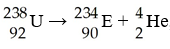In balancing the nuclear reaction [see image], the identity of element E is ________.
A) Pu
B) Np
C) U
D) Pa
E) Th

E

73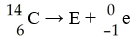What is the identity of element E in the nuclear reaction [see image]?
A) B
B) C
C) O
D) N
E) none of the above

D

74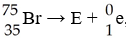In balancing the nuclear reaction [see image], the identity of element E is ________.
A) Kr
B) Br
C) U
D) Se
E) none of the above

D

75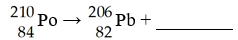This reaction is an example of ________.

A) alpha decay
B) beta emission
C) gamma emission
D) positron emission
E) electron capture

A

76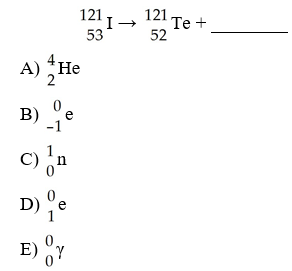The missing product from this reaction is ________.

D

77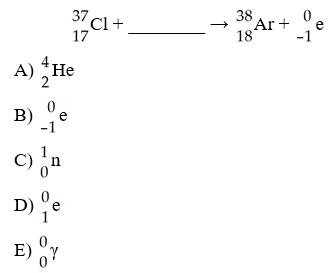The missing reactant from this reaction is ________.

C

78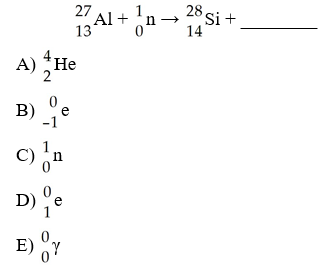The missing product from this reaction is ________.

B

79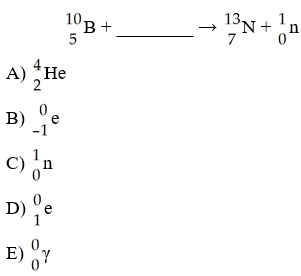The missing reactant from this reaction is ________.

A

80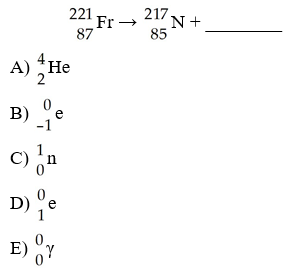The missing product from this reaction is ________.

A

81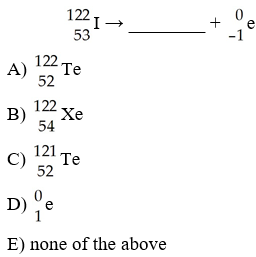The missing product from this reaction is ________.

B

82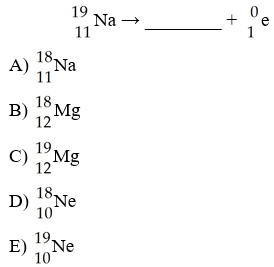The missing product from this reaction is ________.

E

83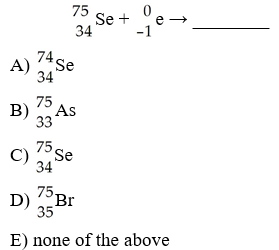The missing product from this reaction is ________.

B

84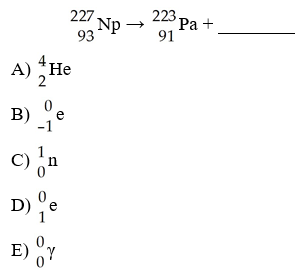The missing product from this reaction is ________.

A

85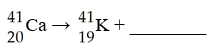This reaction is an example of ________.

A) alpha decay
B) beta decay
C) positron decay
D) electron capture
E) gamma emission

C

86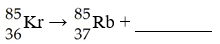This reaction is an example of ________.

A) alpha decay
B) beta decay
C) positron decay
D) electron capture
E) gamma emission

B

87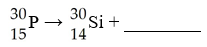This reaction is an example of ________.

A) alpha decay
B) beta decay
C) positron decay
D) electron capture
E) gamma emission

C

88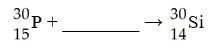This reaction is an example of ________.

A) alpha decay
B) beta decay
C) positron decay
D) electron capture
E) gamma emission

D

89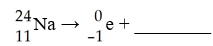The missing product in this reaction would be found in which group of the periodic table?

A) 1A
B) 2A
C) 3A
D) 8A
E) 7A

B

90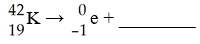The missing product in this reaction combines with oxygen to form a compound with the formula ________.

A) M2O
B) MO
C) M
D) M2O3
E) M3O2

B

91

Radium undergoes alpha decay. The product of this reaction also undergoes alpha decay. What is the product of this second decay reaction?
A) Po
B) Rn
C) U
D) Th
E) Hg

A

92

41Ca decays by electron capture. The product of this reaction undergoes alpha decay. What is the product of this second decay reaction?
A) Ti
B) Ca
C) Ar
D) Cl
E) Sc

D

93

What is the mass number of a neutron?
A) 2
B) 1
C) 3
D) 4
E) 0

B

94

Nuclei above the belt of stability can lower their neutron-to-proton ratio by ________.
A) beta emission
B) gamma emission
C) positron emission
D) electron capture
E) Any of the above processes will lower the neutron-to-proton ratio.

A

95

What is the largest number of protons that can exist in a nucleus and still be stable?
A) 206
B) 50
C) 92
D) 83
E) 84

D

96

The three radioactive series that occur in nature end with what element?
A) Bi
B) U
C) Po
D) Pb
E) Hg

D

97

The largest number of stable nuclei have an ________ number of protons and an ________ number of neutrons.
A) even, even
B) odd, odd
C) even, odd
D) odd, even
E) even, equal

A

98

In the nuclear transmutation represented by 16 8O(p, α) 13 7N, the emitted particle is ________.
A) a beta particle
B) an alpha particle
C) a proton
D) a positron
E) a neutron

B

99

Bombardment of uranium-235 with a neutron generates tellurium-135, 3 neutrons, and ________.
A) zirconium-98
B) krypton-101
C) krypton-103
D) strontium-99
E) zirconium-99

A

100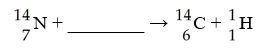The reaction shown below is responsible for creating 14C in the atmosphere. What is the bombarding particle?

A) alpha particle
B) electron
C) neutron
D) positron
E) proton

C

101

How many neutrons are emitted when a californium-249 nucleus (Z=98) is bombarded with a carbon-12 nucleus to produce a 257 104Rf nucleus?
A) one
B) three
C) two
D) four
E) zero

D

102

How many neutrons are emitted when a californium-249 nucleus (Z=98) is bombarded with a nitrogen-15 nucleus to produce a 260 105Db nucleus?
A) two
B) three
C) four
D) one
E) zero

C

103

What order process is radioactive decay?
A) zeroth
B) first
C) second
D) third
E) fourth

B

104

Due to the nature of the positron, ________ is actually detected in positron emission tomography.
D) x-ray emission
E) neutron emission

C

105

The mass of a proton is 1.00728 amu and that of a neutron is 1.00867 amu. What is the mass defect (in amu) of a 60 27Co nucleus? (The mass of a cobalt-60 nucleus is 59.9338 amu.)
A) 27.7830
B) 0.5489
C) 0.5405
D) 0.0662
E) 0.4827

B

106

What is the typical percent of uranium-235 in the enriched UO2 pellets used in nuclear reactors?
A) 0.7
B) 1
C) 3
D) 10
E) 14

C

107

On average, ________ neutrons are produced by every fission of a uranium-235 nucleus.
A) 4
B) 3.5
C) 1
D) 2.4
E) 2

D

108

What drives the turbine in a nuclear power plant?
A) the moderator
B) steam
C) the control rods
D) the primary coolant
E) UF6 gas

B

109

Who is credited with first achieving fission of uranium-235?
A) Fermi
B) Rutherford
C) Curie
D) Dalton

A

110

When ionizing radiation enters the body, what is the predominant free radical produced?
A) H
B) H3O
C) protein
D) OH
E) H2O

D

111

A ________ is a highly reactive substance that contains one or more unpaired electrons.
A) cation
C) anion
D) protein

B

112

What happens to the mass number and the atomic number of an element when it undergoes alpha decay?
A) The mass number decreases by 4 and the atomic number decreases by 2.
B) The mass number does not change and the atomic number increases by 1.
C) The mass number increases by 4 and the atomic number does not change.
D) The mass number increases by 2 and the atomic number decreases by 1.
E) The mass number does not change and the atomic number increases by 2.

A

113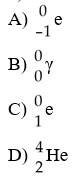Which one of the following is a correct representation of beta particle?

A

114

Which one of the following processes results in a decrease in the number of neutrons?
A) alpha emission
B) gamma emission
C) positron emission
D) corrosion
E) electron capture

A

115

In what type of radioactive decay does the atomic number of the product decrease by one?
A) positron emission
B) corrosion
C) alpha
D) beta
E) gamma

A

116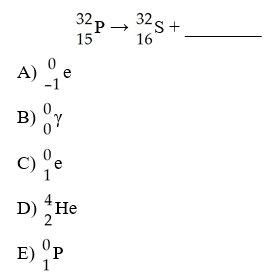What is the missing product from this reaction?

A

117

When an atom of an element undergoes beta decay, its proton count will change by ________ and its neutron count will change by ________.
A) +1, -1
B) 0, 0
C) -1, +1
D) -2, -2
E) -1, -1

A

118

What is the missing product from this reaction?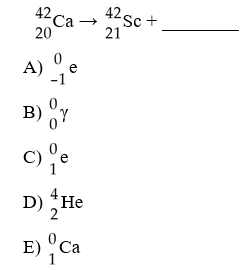A

119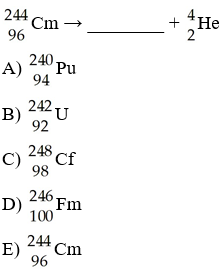What is the missing product from this reaction?

A

120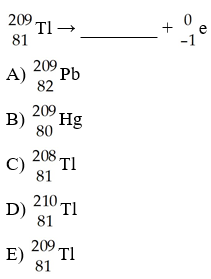What is the missing product from this reaction?

A

121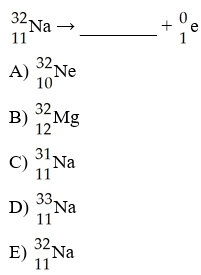What is the missing product from this reaction?

A

122

The product of the nuclear reaction in which 40Ar is subjected to neutron capture followed by alpha emission is ________.
A) 37S
B) 41Ar
C) 36S
D) 45Ca
E) 35Ar

A

123

The half-life of cobalt-60 is 5.20 yr. How many milligrams of a 2.000-mg sample remain after 9.50 years?
A) 0.565
B) 7.03 × 10-22
C) 7.076
D) 1.095
E) 1.435

A

124

What percentage of a radioactive sample remains after 175.0 yr if it has a of 28.8 years?
A) 84.8
B) 0.230
C) 16.5
D) 6.08
E) 1.48

E

125

What percentage of a sample remains after 50.0 min if it has a half-life of 20.4 min.?
A) 8.62
B) 66.5
C) 40.8
D) 18.3
E) 2.45

D

126

A rock contains 0.153 mg of lead-206 for each milligram of uranium-238. The half-life for the decay of uranium-238 to lead-206 is 4.5 × 109 yr. The rock was formed ________ years ago.
A) 6.89 × 108
B) 5.60 × 108
C) 7.33 × 108
D) 1.06 × 109
E) 8.08 × 108

D

127

131I has a half-life of 8.04 days. Assuming you start with a 1.03 mg sample of 131I, how many mg will remain after 13.0 days?
A) 0.326
B) 0.268
C) 0.422
D) 0.0781
E) 0.336

E

128

The decay of a radionuclide with a half-life of 3.3 × 105 years has a rate constant (in yr-1) equal to ________.
A) 4.8 × 105
B) 2.1 × 10-6
C) 4.2 × 10-6
D) 2.8 × 103
E) 5.9 × 10-8

B

129

What is the age in years of a mineral sample that has a mass ratio of 40Ar to 40K of 0.330? Potassium-40 decays to argon-40 with a half-life of 1.27 × 109 yr.
A) 3.85 × 109
B) 5.23 × 108
C) 1.77 × 109
D) 3.62 × 108
E) 2.55 × 109

B

130

If we start with 1.000 g of strontium-90, 0.805 g will remain after 9.00 yr. This means that the half-life of strontium-90 is ________ yr.
A) 7.74
B) 11.2
C) 28.8
D) 7.25
E) 41.6

C

131

If we start with 1.000 g of cobalt-60, 0.400 g will remain after 7.00 yr. This means that the half-life of cobalt-60 is ________ yr.
A) 12.1
B) 17.5
C) 2.80
D) 5.30
E) 7.65

D

132

A freshly prepared sample of curium-243 undergoes 3312 disintegrations per second. After 8.00 yr, the activity of the sample declines to 2591 disintegrations per second. What is the half-life in years of curium-243?
A) 22.6
B) 6.26
C) 10.2
D) 1.36
E) 32.6

A

133

The beta decay of cesium-137 has a half-life of 30.0 years. How many years must pass to reduce a 30 mg sample of cesium 137 to 5.2 mg?
A) 76 years
B) -76 years
C) 38 years
D) 0.040 years
E) 25 years

A

134

The half-life of 218Po is 3.1 minutes. How much of a 170 gram sample remains after 0.64 hours?
A) 0.032 g
B) 150 g
C) 31 g
D) 4.7 × 10-10 g
E) None of the original sample will remain.

A

135

Cesium-131 has a half-life of 9.7 days. What percent of a cesium-131 sample remains after 62 days?
A) 1.2 %
B) 100 %
C) 0 %
D) 99 %
E) 62%

A

136

The half-life of carbon-11 is 20.3 minutes. How much of a 100.0 mg sample remains after 1.6 hours?
A) 3.77 mg
B) 0.0377 mg
C) 94.7 mg
D) 1.75 × 10-89 mg
E) 99.9 mg

A

137

210Pb has a half-life of 22.3 years and decays to produce 206Hg. If you start with of 210Pb, how many grams of 206Hg will you have after 15.8 years?
A) 4.60 g
B) 2.83 g
C) 9.20 g
D) 2.30 g
E) 12.5 g

D

138

Carbon-11 decays by positron emission. The decay occurs with a release of 2.87 × 1011 J per mole of carbon-11. What mass (g) is converted to energy when 5.00 g of carbon-11 undergoes this radioactive decay?
A) 1.45 × 10-6
B) 4.35 × 105
C) 6.90 × 102
D) 1.45 × 10-3
E) 1.59 × 10-2

D

139

How much energy (in J) is produced when 0.067 g of matter is converted to energy?
A) 6.0 × 1018
B) 6.0 × 1012
C) 2.0 × 104
D) 6.0 × 1015
E) 2.0 × 107

B

140

The mass of a proton is 1.673 × 10-24g. The mass of a neutron is 1.675 × 10-24g. The mass of the nucleus of an 59Fe atom is 9.787 × 10-23 g. What is the nuclear binding energy (in J) for A 59Fe nucleus? (c = 3.00 x 108m/s)
A) 8.13 × 10-11 J
B) 2.71 × 10-19 J
C) 8.13 × 10-8 J
D) 4.00 × 10-9 J
E) -9.74 × 10-10 J

A

141

The mass of a proton is 1.00728 amu and that of a neutron is 1.00867 amu. What is the binding energy (in J) of a 62Co nucleus?
(The mass of a cobalt-62 nucleus is 61.9341 amu. The speed of light is 3.00 x 108m/s.)
A) 8.46 × 10-11 J
B) 8.46 × 10-19 J
C) 4.15 × 10-9 J
D) 5.09 × 1016 J
E) -1.12 × 10-9 J

A

142

The mass of a proton is 1.00728 amu and that of a neutron is 1.00867 amu. What is the binding energy per nucleon (in J) of a 59Co nucleus?
(The mass of a cobalt-59 nucleus is 58.9332 amu. The speed of light is 3.00 x 108m/s.)
A) 8.46 × 10-12 J
B) 8.46 × 1013 J
C) 4.15 × 1034 J
D) 5.09 × 1012 J
E) -1.12 × 1010 J

A

143

The mass of a proton is 1.00728 amu and that of a neutron is 1.00867 amu. What is the mass defect (in amu) of a 57Ni nucleus?
(The mass of a nickel-57 nucleus is 56.938 amu. )
A) 0.5155 amu
B) 28.76 amu
C) -0.4932 amu
D) 0.5141 amu
E) 1.031 amu

A

144

What happens in the nucleus of an atom that undergoes positron emission?

A proton is converted to a neutron and a positron.

145

What is the predominant isotope of uranium?

238U

146

What happens to the atomic mass number and the atomic number of a radioisotope when it undergoes alpha emission?

The mass number drops by 4 and the atomic number decreases by 2.

147

What are beta particles?

high speed electrons emitted by an unstable nucleus

148

4 2He represents ________.

an alpha particle

149

What isotope of what element is produced if uranium-238 undergoes alpha decay?

150

Stable nuclei with low atomic numbers, up to ________, have a neutron to proton ratio of approximately 1:1.

20

151

The first nuclear transmutation resulted in the conversion of nitrogen-14 to ________.

oxygen-17

152

Conversion of one nucleus into another was first demonstrated in 1919 by ________.

Rutherford

153

The initial element used to make cobalt-60 for cancer radiation therapy is ________.

iron; Fe

154

What is the rate constant for the decay of some unknown radioactive compound if the half-life for the beta decay is 1.3 × 109 years?

5.3 × 10-10 year-1

155

Becquerel

156

Carbon-11, fluorine-18, oxygen-15 and nitrogen-13 are all used in the clinical diagnostic technique known as ________.

positron emission tomography; PET

157

The conversion of matter to energy and mass loss occurs in ________ reactions?

nuclear

158

Control rods in a nuclear reactor are composed of boron and ________.

an alloy of silver, indium, and cadmium

159

The amount of fissionable material necessary to maintain a chain reactions is called the ________.

critical mass

160

What was the purpose of the Manhattan project?

to build a bomb based on nuclear fission

161

When living tissue is irradiated most of the energy is absorbed by ________.

water

162

The relative biological effectiveness (RBE) values of beta rays, gamma rays, and alpha rays are ________, respectively.

1,1,10

163

The major type of cancer caused by radiation is ________.

leukemia

164

Radioactive seeds that are implanted into a tumor are coated with ________ to stop alpha and beta ray penetration.

platinum

165

Gamma radiation only changes the atomic number but not the mass number of a nucleus.

false

166

Positron emission causes an increase of one in the atomic number.

false

167

The neutron/proton ratio of stable nuclei increases with increasing atomic number.

true

168

Charged particles are accelerated because the faster they move there is a greater chance of producing a nuclear reaction.

true

169

Radioactive decay is a first order kinetic process.

true

170

In radioactive dating, the ratio of carbon-12 to carbon-14 is related to the time of death of the animal or plant under investigation.

false

171

In the formula k = 0.693/t1/2, k is the decay constant.

true

172

The energy produced by the sun is the result of nuclear fusion.

true

173

The SI unit of an absorbed dose of radiation is the gray.

true

174

The relative biological effectiveness (RBE) is tenfold greater for gamma radiation than for alpha radiation.

false

175

Electrons do not exist in the nucleus, yet beta emission is ejection of electrons from the nucleus. How does this happen?

A neutron breaks apart to produce a proton and an electron in the nucleus. The proton remains in the nucleus and the electron is ejected.

176

List the common particles and their symbols used in descriptions of radioactive decay and nuclear transformations.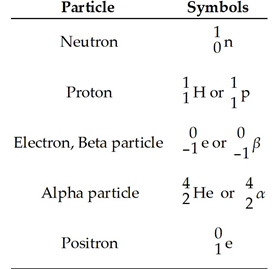177

When an isotope undergoes electron capture, what happens to the captured electron?

It combines with a proton in the nucleus to form a neutron.

178

The use of radioisotopes in tracing metabolism is possible because ________.

all isotopes of an element have identical chemical properties